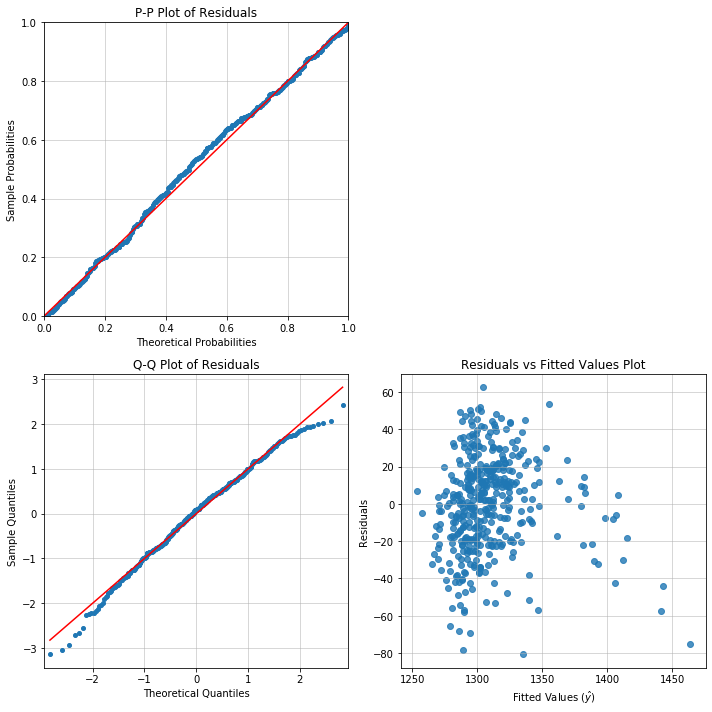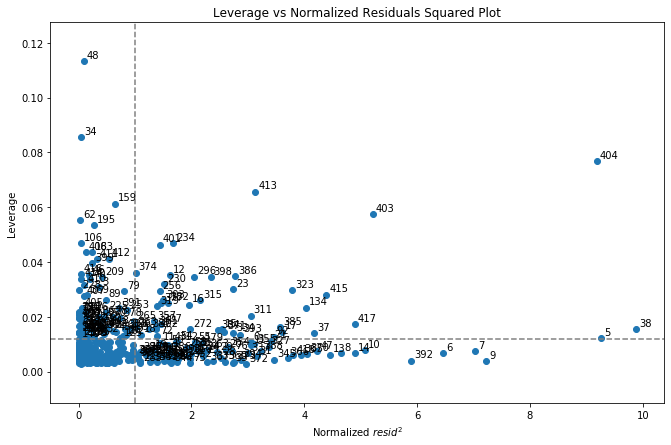10 MINUTES TO APPELPY

In :
import pandas as pd
import numpy as np
import statsmodels.api as sm  # for datasets
import matplotlib.pyplot as plt
%matplotlib inline


This is a whirlwind tour of Appelpy's functionality:

• Exploratory data analysis
• Model estimation
• Model diagnostics

Note: this notebook was run using Appelpy v0.4.2 and the latest versions of its dependencies.

# Set up model dataset¶

Let's explore the functionality of Appelpy using one of the most prolific datasets in econometrics: The California Test Score Data Set (Caschool).

Read the dataset with Pandas and obtain the data via a Statsmodels method.

In :
# Load data
df = sm.datasets.get_rdataset('Caschool', 'Ecdat').data
# Add together the two test scores (total score)
df['scrtot'] = df['readscr'] + df['mathscr']

In :
df.head()

Out:
distcod county district grspan enrltot teachers calwpct mealpct computer testscr compstu expnstu str avginc elpct readscr mathscr scrtot
0 75119 Alameda Sunol Glen Unified KK-08 195 10.900000 0.510200 2.040800 67 690.799988 0.343590 6384.911133 17.889910 22.690001 0.000000 691.599976 690.000000 1381.599976
1 61499 Butte Manzanita Elementary KK-08 240 11.150000 15.416700 47.916698 101 661.200012 0.420833 5099.380859 21.524664 9.824000 4.583333 660.500000 661.900024 1322.400024
2 61549 Butte Thermalito Union Elementary KK-08 1550 82.900002 55.032299 76.322601 169 643.599976 0.109032 5501.954590 18.697226 8.978000 30.000002 636.299988 650.900024 1287.200012
3 61457 Butte Golden Feather Union Elementary KK-08 243 14.000000 36.475399 77.049202 85 647.700012 0.349794 7101.831055 17.357143 8.978000 0.000000 651.900024 643.500000 1295.400024
4 61523 Butte Palermo Union Elementary KK-08 1335 71.500000 33.108601 78.427002 171 640.849976 0.128090 5235.987793 18.671329 9.080333 13.857677 641.799988 639.900024 1281.700012

# Inspect data¶

Now we have the data, let's find out more about the distributions of some of the key variables before modelling.

Appelpy has an eda module (exploratory data analysis) with handy methods for getting stats about a dataset.

In :
from appelpy.eda import statistical_moments


Get all the statistical moments in one place:

1. Mean
2. Variance
3. Skewness (Fisher as default)
4. Kurtosis
In :
statistical_moments(df)

Out:
mean var skew kurtosis
distcod 67472.8 1.20201e+07 -0.0307844 -1.09626
enrltot 2628.79 1.53124e+07 2.87017 10.2004
teachers 129.067 35311.2 2.93254 11.219
calwpct 13.246 131.213 1.68306 4.58959
mealpct 44.7052 735.678 0.183954 -0.999802
computer 303.383 194782 2.87169 10.7729
testscr 654.157 363.03 0.0916151 -0.254288
compstu 0.135927 0.00421926 0.922369 1.43113
expnstu 5312.41 401876 1.0679 1.87571
str 19.6404 3.57895 -0.0253655 0.609597
avginc 15.3166 52.2135 2.21516 6.53212
elpct 15.7682 334.375 1.4268 1.4354
readscr 654.97 404.331 -0.0583962 -0.358049
mathscr 653.343 351.72 0.255082 -0.159811
scrtot 1308.31 1452.12 0.0916149 -0.254288

# Model estimation¶

At the core of Appelpy is modelling phenomena in datasets.

The linear_model module contains classes for modelling data, e.g. Ordinary Least Squares (OLS).

In :
from appelpy.linear_model import OLS


Here's a recipe for estimating a linear model.

1. Make two lists – y_list and X_list – for your dependent variable (y) and independent variables (X).
In :
y_list = ['scrtot']
X_list = ['avginc', 'str', 'enrltot', 'expnstu']

1. Fit a model in one line, by passing the model dataframe and two lists as inputs to the OLS object.
In :
model_nonrobust = OLS(df, y_list, X_list).fit()

1. Get the key model results in the model_selection_stats attribute, including the Root Mean Square Error (Root MSE).
In :
model_nonrobust.model_selection_stats

Out:
{'root_mse': 25.85923055850994,
'r_squared': 0.5438972040191434,
'r_squared_adj': 0.5395010324916171,
'aic': 3929.1191674301117,
'bic': 3949.320440986499}

Get the Statsmodels summary via the results_output attribute...

In :
model_nonrobust.results_output

Out:
Dep. Variable: R-squared: scrtot 0.544 OLS 0.540 Least Squares 123.7 Tue, 18 Feb 2020 2.05e-69 19:28:04 -1959.6 420 3929. 415 3949. 4 nonrobust
coef std err t P>|t| [0.025 0.975] 1312.9203 27.833 47.171 0.000 1258.208 1367.632 3.8640 0.185 20.876 0.000 3.500 4.228 -1.3954 0.893 -1.563 0.119 -3.150 0.359 -0.0016 0.000 -4.722 0.000 -0.002 -0.001 -0.0061 0.003 -2.316 0.021 -0.011 -0.001
 Omnibus: Durbin-Watson: 7.321 0.763 0.026 7.438 -0.326 0.0243 2.969 139000

Warnings:
 Standard Errors assume that the covariance matrix of the errors is correctly specified.
 The condition number is large, 1.39e+05. This might indicate that there are
strong multicollinearity or other numerical problems.

...but now it's finally easy to get the standardized model results via the results_output_standardized attribute.

In :
model_nonrobust.results_output_standardized
# This is a Styler object; add .data to access the underlying Pandas dataframe

Out:
Unstandardized and Standardized Estimates
coef t P>|t| coef_stdX coef_stdXy stdev_X
scrtot
avginc +3.8640 +20.876 0.000 +27.9209 +0.7327 7.2259
str -1.3954 -1.563 0.119 -2.6399 -0.0693 1.8918
enrltot -0.0016 -4.722 0.000 -6.3035 -0.1654 3913.1050
expnstu -0.0061 -2.316 0.021 -3.8364 -0.1007 633.9371

The output is similar to that of Stata's listcoef command.

# Model diagnostics¶

Call the model object's diagnostic_plot method to bring up many visual regression diagnostics:

• pp_plot: P-P plot
• qq_plot: Q-Q plot
• rvp_plot: Residuals vs Predicted Values plot
• rvf_plot: Residuals vs Fitted Values plot

Appelpy's diagnostics module has a suite of classes and functions for regression diagnostics:

• If the visual diagnostics are not enough, then do formal tests by calling other methods such as heteroskedasticity_test.
• Are there observations that are stinking up the model? Pass the model object to the BadApples class and examine the influential points, outliers and high-leverage points.

## Plots¶

Here's a recipe for a 2x2 set of diagnostic plots.

In :
fig, axarr = plt.subplots(2, 2, figsize=(10, 10))
model_nonrobust.diagnostic_plot('pp_plot', ax=axarr)
model_nonrobust.diagnostic_plot('qq_plot', ax=axarr)
model_nonrobust.diagnostic_plot('rvf_plot', ax=axarr)
axarr[0, 1].axis('off')
plt.tight_layout()Oh dear. The model specified has particular problems with test scores of 1350 and above.

There appears to be non-constance variance in the RVF plot.

Perhaps there is heteroskedasticity too, but we could do more specific tests there.

## Heteroskedasticity¶

In :
from appelpy.diagnostics import heteroskedasticity_test


Call one of a few heteroskedasticity tests, e.g.:

• Breusch-Pagan (default of Stata's hettest command)
• Bresuch-Pagan studentized (default of R's bptest command)
• White (Stata's hettest, white command)
In :
bp_stats = heteroskedasticity_test('breusch_pagan', model_nonrobust)
print('Breusch-Pagan test :: {}'.format(bp_stats['distribution'] + '({})'.format(bp_stats['nu'])))
print('Test statistic: {:.4f}'.format(bp_stats['test_stat']))
print('Test p-value: {:.4f}'.format(bp_stats['p_value']))

Breusch-Pagan test :: chi2(1)
Test statistic: 2.5579
Test p-value: 0.1097

In :
bps_stats = heteroskedasticity_test('breusch_pagan_studentized', model_nonrobust)
print('Breusch-Pagan test (studentized) :: {}'.format(bps_stats['distribution'] + '({})'.format(bps_stats['nu'])))
print('Test statistic: {:.4f}'.format(bps_stats['test_stat']))
print('Test p-value: {:.4f}'.format(bps_stats['p_value']))

Breusch-Pagan test (studentized) :: chi2(1)
Test statistic: 11.3877
Test p-value: 0.0225


The studentized Breusch-Pagan test is seen as more robust and is showing a significant difference from the initial hypothesis of homoskedasticity, so let's fit a model with heteroskedastic-robust standard errors (HC1 errors).

In :
model_hc1 = OLS(df, y_list, X_list, cov_type='HC1').fit()

In :
model_hc1.results_output

Out:
Dep. Variable: R-squared: scrtot 0.544 OLS 0.540 Least Squares 78.60 Tue, 18 Feb 2020 1.36e-49 19:28:05 -1959.6 420 3929. 415 3949. 4 HC1
coef std err z P>|z| [0.025 0.975] 1312.9203 29.364 44.711 0.000 1255.367 1370.474 3.8640 0.240 16.119 0.000 3.394 4.334 -1.3954 0.932 -1.498 0.134 -3.221 0.430 -0.0016 0.000 -5.794 0.000 -0.002 -0.001 -0.0061 0.003 -2.166 0.030 -0.012 -0.001
 Omnibus: Durbin-Watson: 7.321 0.763 0.026 7.438 -0.326 0.0243 2.969 139000

Warnings:
 Standard Errors are heteroscedasticity robust (HC1)
 The condition number is large, 1.39e+05. This might indicate that there are
strong multicollinearity or other numerical problems.

What are the significant regressors (independent variables) now?

In :
model_hc1.significant_regressors(0.05)

Out:
['avginc', 'enrltot', 'expnstu']

The str (student-teacher ratio) and expnstu regressors specifically relate to investment in students. We can do a Wald test to test whether the two regressors together are significantly different from 0.

In :
from appelpy.diagnostics import wald_test

In :
wald_stats = wald_test(model_hc1, ['str', 'expnstu'])
print('Wald test :: {}'.format(wald_stats['distribution'] + '({})'.format(wald_stats['nu'])))
print('Test statistic: {:.4f}'.format(wald_stats['test_stat']))
print('Test p-value: {:.4f}'.format(wald_stats['p_value']))

Wald test :: chi2(2)
Test statistic: 4.7352
Test p-value: 0.0937


It's unsurprising that the standard errors for some of the variables are higher for HC1 errors compared to non-robust errors.

In :
pd.concat([pd.Series(model_nonrobust.results.bse, name='std_error_nonrobust'),
pd.Series(model_hc1.results.bse, name='std_error_hc1')], axis='columns')

Out:
std_error_nonrobust std_error_hc1
const 27.833430 29.364467
avginc 0.185093 0.239715
str 0.892703 0.931541
enrltot 0.000341 0.000278
expnstu 0.002613 0.002794

## Unusual observations: leverage, outliers and influence¶

Let's inspect observations that have:

• High influence
• High leverage
• Outlier value
In :
from appelpy.diagnostics import BadApples


Set up an instance of BadApples with the model object.

In :
bad_apples = BadApples(model_hc1).fit()


Let's plot the leverage values against the normalized residuals squared.

Observations in the top-right quadrant have leverage values and residual values higher than the average (i.e. they are the most influential in the model).

In :
fig, ax = plt.subplots(figsize=(11,7))
bad_apples.plot_leverage_vs_residuals_squared(annotate=True)
plt.show()### Leverage¶

The leverage values are stored in the measures_leverage attribute.

In :
bad_apples.measures_leverage.sort_values(ascending=False).head()

Out:
48     0.113247
34     0.085633
404    0.076762
413    0.065741
159    0.061353
Name: leverage, dtype: float64

Let's look at the five observations with highest leverage.

In :
bad_apples.show_extreme_observations().loc[[48,34,404,413,159]]

Out:
scrtot avginc str enrltot expnstu
48 1261.099976 12.109128 19.017494 27176 5864.366211
34 1252.200012 11.722225 21.194069 25151 5117.039551
404 1387.900024 55.327999 16.262285 1059 6460.657227
413 1398.200012 50.676998 15.407042 687 7217.263184
159 1294.500000 14.298300 20.291372 21338 5123.474121
In :
model_hc1.X_standardized.loc[[48,34,404,413,159]]

Out:
avginc str enrltot expnstu
48 -0.443884 -0.329278 6.273077 0.870684
34 -0.497428 0.821246 5.755585 -0.308182
404 5.537230 -1.785664 -0.401163 1.811299
413 4.893572 -2.237740 -0.496228 3.004803
159 -0.140922 0.344087 4.781167 -0.298032

These high-leverage points have at least one independent variable with a value > 4 standard deviations away from the mean.

### Outliers¶

The BadApples object calculates heuristics for determining whether values of measures are 'extreme'.

The extreme indices are stored in attributes, e.g. outliers stored in indices_outliers.

In :
bad_apples.indices_outliers

Out:
[5, 6, 7, 8, 9, 10, 13, 14, 37, 38, 47, 134, 370, 392, 403, 404, 415, 417]

The heuristic for outliers: an observation is an outlier if either the absolute value of the standardized residual or absolute value of the studentized residual is higher than 2.

In :
print(f"Proportion of observations as outliers: {len(bad_apples.indices_outliers) / len(df):.4%}")

Proportion of observations as outliers: 4.2857%


By that heuristic, we'd expect (by design) no more than around 5% of the observations to be viewed as outliers.

### Influence¶

Also consider a range of influence measures, e.g. Cook's distance, dffits and dfbeta.

In :
bad_apples.measures_influence.sort_values('cooks_d', ascending=False).head()

Out:
dfbeta_const dfbeta_avginc dfbeta_str dfbeta_enrltot dfbeta_expnstu cooks_d dffits_internal dffits
404 0.010742 -0.811324 0.068295 0.061925 0.039761 0.152768 -0.873981 -0.882753
403 0.024082 -0.529623 0.016131 0.031978 0.026944 0.063718 -0.564440 -0.567338
413 0.074297 -0.373064 0.013021 0.033955 -0.099557 0.044116 -0.469661 -0.470876
38 -0.277356 -0.193382 0.217223 -0.136237 0.315817 0.030955 -0.393412 -0.397701
415 -0.157629 0.099707 0.045684 -0.026836 0.249169 0.025414 0.356468 0.357936

# Summary¶

• Load your dataset and do your pre-processing with Pandas.
• Appelpy eda: inspect your model dataset with handy functions, e.g. statistical moments.
• Appelpy modelling: linear_model contains classes such as OLS and WLS for model estimation. Use a short recipe for each model and access model estimates, e.g. results_output and results_output_standardized.
• Appelpy diagnostics: check whether the model estimates are valid with diagnostics, e.g. diagnostic plots, statistical tests and BadApples for influence analysis.

Other features worth checking out:

• Logistic regression (under discrete_model)
• DummyEncoder and InteractionEncoder classes